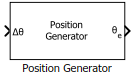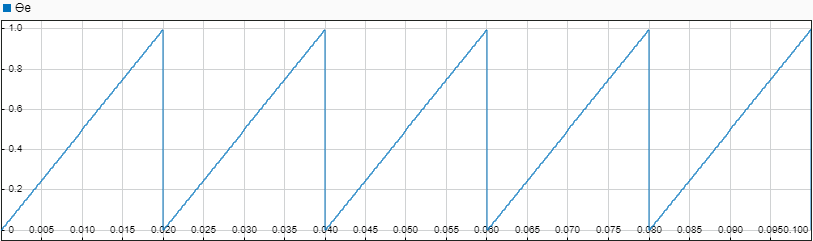# Position Generator

Generate position ramp of fixed frequency

• Library:
• Motor Control Blockset / Controls / Control Reference

•## Description

The Position Generator block generates a position ramp signal (with a frequency that is identical to that of the reference voltage signal) using the position increment value of the reference signal.

We recommend that you use fixed-step discrete solver for this block to enable code generation and ensure accurate simulation.## Ports

### Input

expand all

Position increment value of a fixed frequency reference voltage signal (in either per unit, radians, or degrees). These equations describe how the block computes the position increment:

Data Types: `single` | `double` | `fixed point`

External pulse that resets the position ramp output based on the value of the External reset parameter.

#### Dependencies

To enable this port, set External reset to either `active high resets to zero` or ```active high resets to initial condition```.

Data Types: `single` | `double` | `fixed point`

### Output

expand all

Position or phase value of the reference voltage signal (in either per unit, radians, or degrees).

Data Types: `single` | `double` | `fixed point`

## Parameters

expand all

Unit of the input position increment value and the output reference voltage position.

Output position ramp value (in either per unit, radians, or degrees) at initial time (0 seconds).

Output position ramp value (in either per unit, radians, or degrees) at the time when the block receives an active high external reset pulse. You can reset the output to either zero or to equal the value of the Initial theta output parameter.

## Version History

Introduced in R2020a